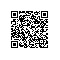# 图表即代码：使用 Diagrams 制作云系统架构原型图

## 二、安装

Diagrams 使用 Graphviz 来渲染图表，在安装 diagrams 之前需要先安装 Graphviz

macOS 用户（如果使用 Homebrew）可以使用 brew install graphviz 的方式来安装 Graphviz

# 使用 pip (pip3)
$pip install diagrams # 使用 pipenv$ pipenv install diagrams

# 使用 poetry
$poetry add diagrams ## 三、快速开始 # diagram.py from diagrams import Diagram from diagrams.alibabacloud.network import SLB from diagrams.alibabacloud.compute import ECS from diagrams.alibabacloud.database import RDS with Diagram("Web Service", show=False): SLB("lb") >> ECS("web") >> RDS("userdb") 执行后，就能生成如下架构图： $ python diagram.py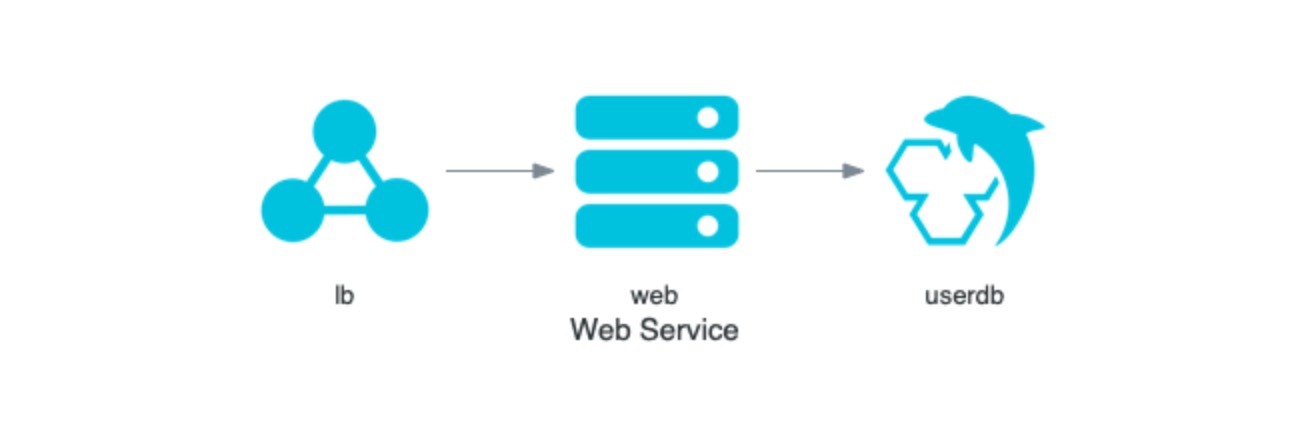## 四、指南

Diagrams 库非常容易掌握，我们仅需要掌握三个概念就能轻松绘制云系统架构图：

• Diagram：这是表示图的最主要的对象，代表一个架构图
• Node：表示一个节点或系统组件，比如快速开始中的SLBECSRDS都是架构图中的节点
• Cluster：表示集群或分组，可将多个节点放到一个集群中

### 4.1 图 Diagram

from diagrams import Diagram
from diagrams.aws.compute import EC2

with Diagram("Simple Diagram"):
EC2("web")

Diagram 类还支持如下参数：

• outformat：指定输出图片的类型，默认是 png，可以是 pngjpgsvgpdf
• show：指定是否显示图片，默认是 False
• graph_attrnode_attredge_attr：指定 Graphviz 属性选项，用来控制图、点、线的样式，详情查看 参考链接

### 4.2 节点 Node

• >>：左节点指向右节点
• <<：右节点指向左节点
• -：节点互相连接，没有方向

from diagrams import Diagram
from diagrams.aws.compute import EC2
from diagrams.aws.database import RDS
from diagrams.aws.network import ELB
from diagrams.aws.storage import S3

with Diagram("Web Services", show=False):
ELB("lb") >> EC2("web") >> RDS("userdb") >> S3("store")
ELB("lb") >> EC2("web") >> RDS("userdb") << EC2("stat")
(ELB("lb") >> EC2("web")) - EC2("web") >> RDS("userdb")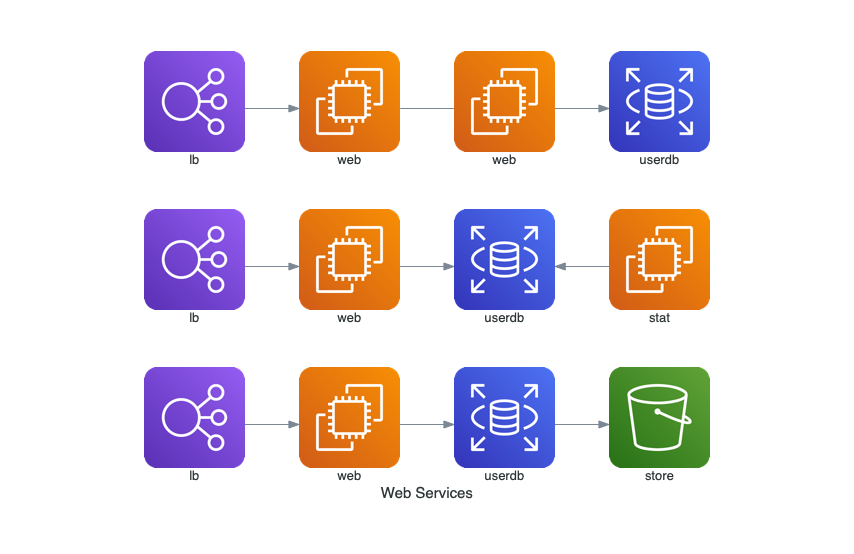Diagrams 不仅支持单个节点的关系建立，还支持一组节点和其他节点的关系建立，使用 list 来表示一组节点。示例如下：

from diagrams import Diagram
from diagrams.aws.compute import EC2
from diagrams.aws.database import RDS
from diagrams.aws.network import ELB

with Diagram("Grouped Workers", show=False, direction="TB"):
ELB("lb") >> [EC2("worker1"),
EC2("worker2"),
EC2("worker3"),
EC2("worker4"),
EC2("worker5")] >> RDS("events")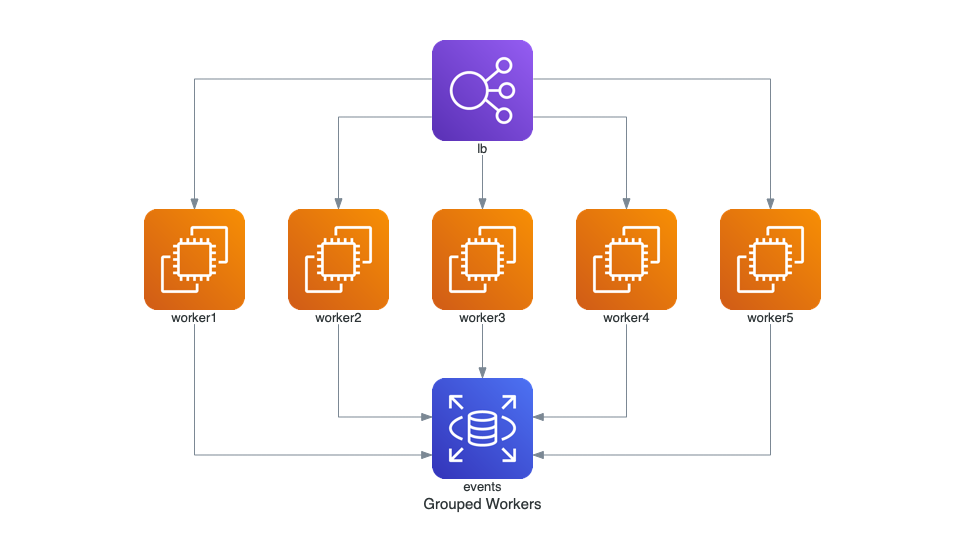### 4.3 集群/组 Cluster

from diagrams import Cluster, Diagram
from diagrams.aws.compute import ECS
from diagrams.aws.database import RDS
from diagrams.aws.network import Route53

with Diagram("Simple Web Service with DB Cluster", show=False):
dns = Route53("dns")
web = ECS("service")

with Cluster("DB Cluster"):
db_master = RDS("master")
db_master - [RDS("slave1"),
RDS("slave2")]

dns >> web >> db_master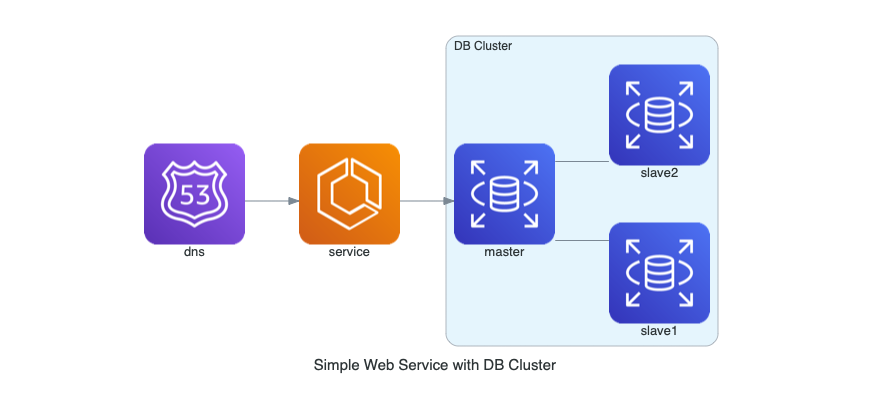Diagrams 还支持嵌套集群，只需嵌套使用 with Cluster() 即可：

from diagrams import Cluster, Diagram
from diagrams.aws.compute import ECS, EKS, Lambda
from diagrams.aws.database import Redshift
from diagrams.aws.integration import SQS
from diagrams.aws.storage import S3

with Diagram("Event Processing", show=False):
source = EKS("k8s source")

with Cluster("Event Flows"):
with Cluster("Event Workers"):
workers = [ECS("worker1"),
ECS("worker2"),
ECS("worker3")]

queue = SQS("event queue")

with Cluster("Processing"):
handlers = [Lambda("proc1"),
Lambda("proc2"),
Lambda("proc3")]

store = S3("events store")
dw = Redshift("analytics")

source >> workers >> queue >> handlers
handlers >> store
handlers >> dw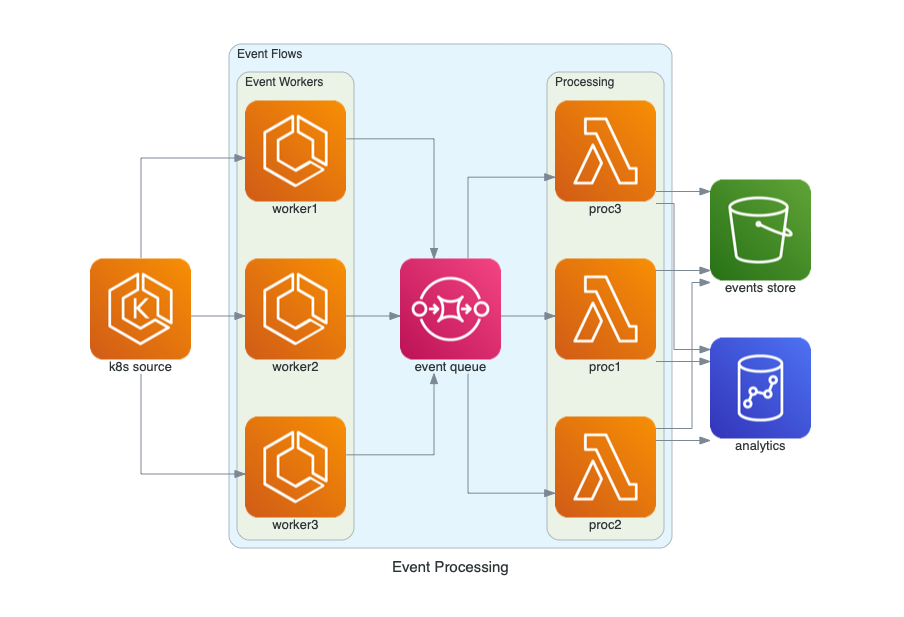## 五、我是如何贡献代码

• 将特定云供应商的 svg 图片转换为 png
• 将特定云供应商的图片调整为圆角图片
• 自动生成节点类代码
• 自动生成文档
• 使用 black 格式化自动生成的代码